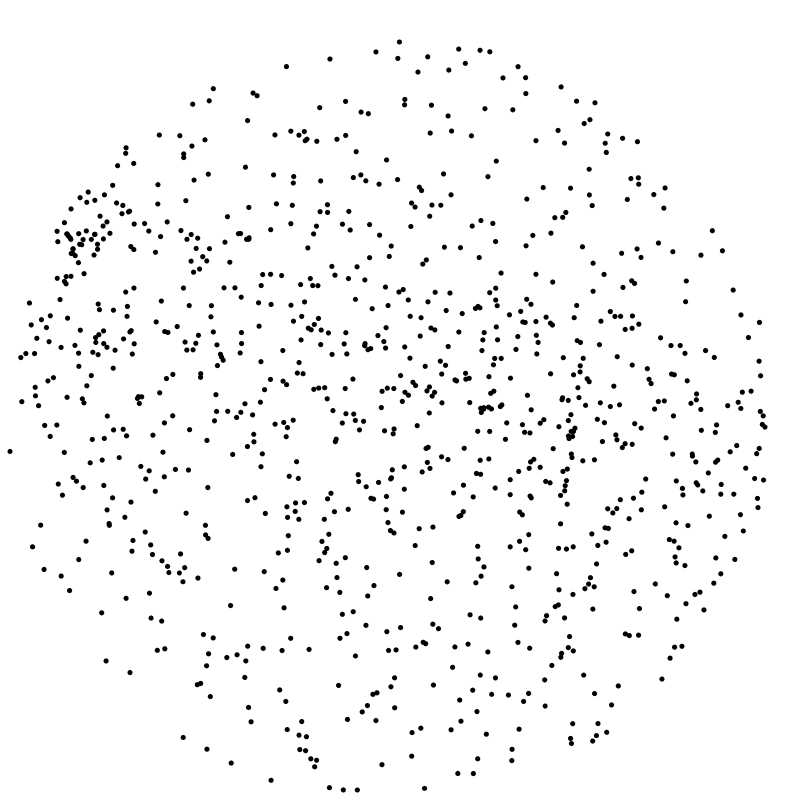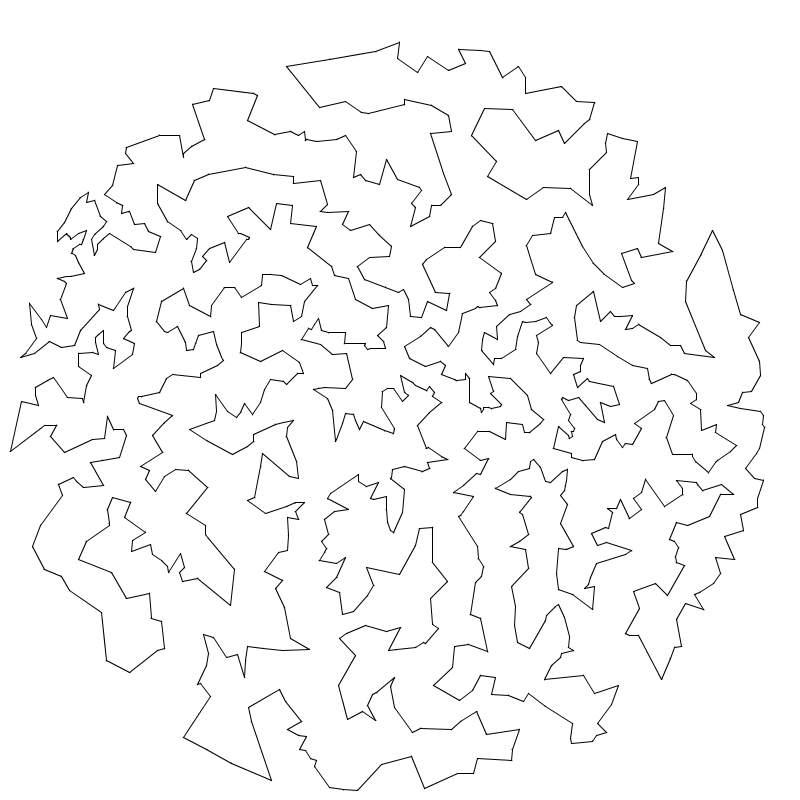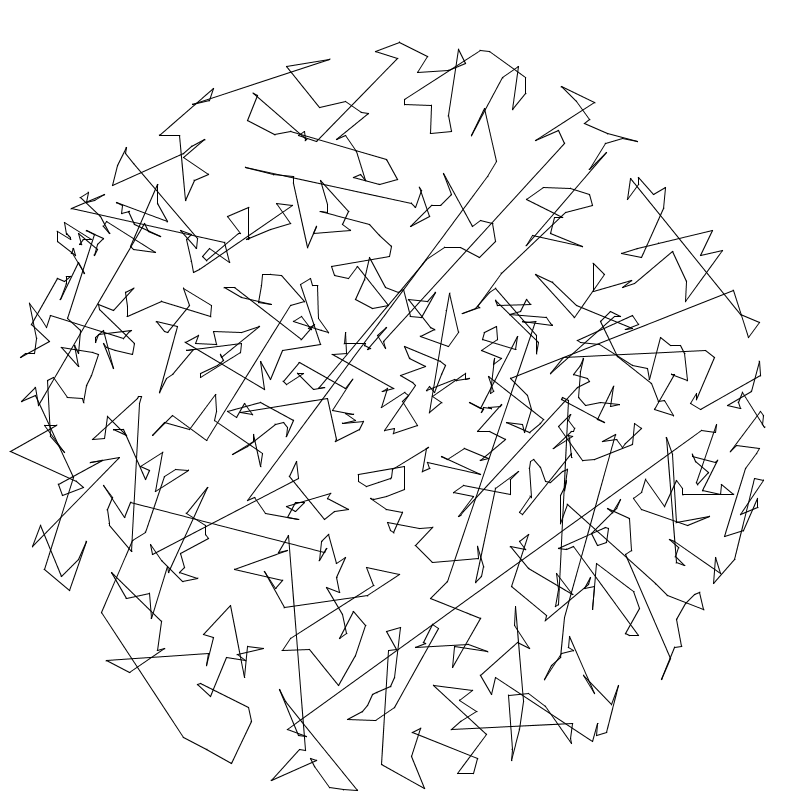COS 126 Traveling Salesperson Problem Programming Assignment Checklist

Given n points in the plane, the goal of a traveling salesperson is to visit all of them (and return to the starting point) while keeping the total length traveled as short as possible. Implement two greedy heuristics to find good (but not optimal) solutions to the traveling salesperson problem (TSP).1,000 points optimal tour

Perspective. The importance of the TSP does not arise from an overwhelming demand of salespeople to minimize their travel length, but rather from a wealth of other applications such as vehicle routing, circuit board drilling, VLSI design, robot control, X-ray crystallography, machine scheduling, and computational biology.

Greedy heuristics. The TSP is a notoriously difficult combinatorial optimization problem. In principle, you can enumerate all possible tours and pick the shortest one; in practice, the number of tours is so staggeringly large (nearly n factorial) that this approach is useless. For large n, no one knows of an efficient method that can find the shortest possible tour for any given set of points. However, many methods have been studied that seem to work well in practice, even though they do not guarantee to produce the best possible tour. Such methods are called heuristics.

Your main task is to implement the nearest neighbor and smallest increase insertion heuristics for building a tour incrementally, one point at a time. Start with a one-point tour (from the first point back to itself), and iterate the following process until there are no points left:

• Nearest neighbor heuristic:  Read in the next point, and add it to the current tour after the point to which it is closest. (If there is more than one point to which it is closest, insert it after the first such point you discover.)

• Smallest increase heuristic:  Read in the next point, and add it to the current tour after the point where it results in the least possible increase in the tour length. (If there is more than one point, insert it after the first such point you discover.)

Point data type. The Point data type represents points in the plane, as described by the following API:

public class Point {
public        Point(double x, double y)  // creates the point (x, y)
public double distanceTo(Point that)     // returns the Euclidean distance between the two points
public   void draw()                     // draws this point to standard drawing
public   void drawTo(Point that)         // draws the line segment between the two points
public String toString()                 // returns a string representation of this point
}

Tour data type. Create a Tour data type that represents the sequence of points visited in a TSP tour. Represent the tour as a circularly linked list of nodes, one for each point in the tour. Each Node contains two references: one to the associated Point and the other to the next Node in the tour. Within Tour.java, define a nested class Node in the standard way:

private class Node {
private Point p;
private Node next;
}
Your Tour data type must implement the following API:

public class Tour {
public        Tour()                                    // creates an empty tour
public        Tour(Point a, Point b, Point c, Point d)  // creates the 4-point tour a->b->c->d->a (for debugging)
public    int size()                                    // returns the number of points in this tour
public double length()                                  // returns the length of this tour
public String toString()                                // returns a string representation of this tour
public   void draw()                                    // draws this tour to standard drawing
public   void insertNearest(Point p)                    // inserts p using the nearest neighbor heuristic
public   void insertSmallest(Point p)                   // inserts p using the smallest increase heuristic

// tests this class by directly calling all constructors and instance methods
public static void main(String[] args)
}

String representation format. The toString() returns a string containing the points, one per line, by (implicitly or explicitly) calling the toString() method for each point, starting with the first point in the tour.

Standard drawing format. The draw() method draws the tour to standard drawing by calling the drawTo() method for each pair of consecutive points. It must produce no other output.

Performance requirements. All instance methods must take time linear (or better) in the number of points currently in the tour. Each constructor must take constant time.

Corner cases. You may assume that no Point argument is null.

Input and testing. The input format begins with two integers width and height, followed by pairs of x- and y-coordinates. All x-coordinates will be real numbers between 0 and width; all y-coordinates will be real numbers between 0 and height. For example, tsp1000.txt contains the following data:

% more tsp1000.txt
800 800
185.0411 457.8824
247.5023 299.4322
701.3532 369.7156
563.2718 442.3282
144.5569 576.4812
535.9311 478.4692
383.8523 458.4757
329.9402 740.9576
...
254.9820 302.2548
After implementing Tour.java, use the client program NearestInsertion.java, which reads the points from standard input; runs the nearest neighbor heuristic; prints the resulting tour, its length, and its number of points to standard output; and draws the resulting tour to standard drawing. SmallestInsertion.java is analogous but runs the smallest increase heuristic.

 % java-introcs NearestInsertion < tsp1000.txt (185.0411, 457.8824) (198.3921, 464.6812) (195.8296, 456.6559) (216.8989, 455.126) (213.3513, 468.0186) (241.4387, 467.413) (259.0682, 473.7961) (221.5852, 442.8863) ... (264.57, 410.328) Tour length = 27868.7106 Number of points = 1000 % java-introcs SmallestInsertion < tsp1000.txt (185.0411, 457.8824) (195.8296, 456.6559) (193.0671, 450.2405) (200.7237, 426.3461) (200.5698, 422.6481) (217.4682, 434.3839) (223.1549, 439.8027) (221.5852, 442.8863) ... (186.8032, 449.9557) Tour length = 17265.6282 Number of points = 1000Analysis.  In your readme.txt, estimate the running time (in seconds) of your program as a function of the number of points n. You may use the client TSPTimer.java help collect data points. (It relies on Stopwatch, which is part of stdlib.jar.) Run the two heuristics for n = 1,000, and repeatedly double n until the execution time exceeds 60 seconds.

Files provided.  The file tsp.zip contains Point.java; the four clients NearestNeighbor.java, SmallestInsertion.java, TSPVisualizer.java and TSPTimer.java; several sample input files; and the readme.txt templates.

Submission.   Submit Tour.java and readme.txt (or just the abbreviated partner readme.txt if your partner submitted the code).

Extra credit. Implement a better TSP heuristic. Here are some ideas. We will award a special prize to whoever finds the shortest tour for a mystery tour with about 1,000 points.

• API requirements. Name your program TSP.java. The only public method in TSP.java is main(), which reads a sequence of points from standard input (in the standard format) and print the resulting tour to standard output, one point per line.

• Performance requirement. It must solve a 1,000-point instance in at most a few seconds and a 10,000-point tour in at most a minute.

• Submission. Submit the following to the TSP leaderboard: TSP.java; any accompanying files; and a leaderboard.txt file, which describes your heuristic.
Copyright © 2000–2016, Robert Sedgewick and Kevin Wayne.